# Introduction to Tensorflow and Linear RegressionThese are our notes and code for the Tensorflow Linear Regression workshop given at UCLA on Friday, 10/13/17. You can also view the PowerPoint slides here.

### Tensorflow Basics

Tensorflow is a graph-based numerical computation library. Complex mathematical operations are described in a graph-like data structure, where nodes in the graph represent the mathematical operations, and connections between nodes represent the flow of data from operation to operation. In Tensorflow, these data are held as tensor objects, which is just a generalization of a matrix.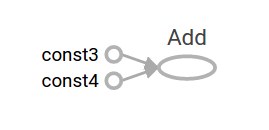We will be using the Python API to access Tensorflow’s data structures and functions, which are implemented in a C++ backend.

A Tensorflow program can be divided into two essential parts:

1. Building a computational graph

2. Launching and running the computational graph

A computational graph is just a series of mathematical operations defined on some data, which we can easily create in Tensorflow. Let’s create our first tensorflow program!

# import our essential libraries
import tensorflow as tf # we can access tensorflow with "tf" now
import numpy as np
import matplotlib.pyplot as plt
%matplotlib inline

a = tf.constant(3.0)
b = tf.constant(4.0)

print(a)
print(b)

Tensor("Const:0", shape=(), dtype=float32)
Tensor("Const_1:0", shape=(), dtype=float32)


Printing out the nodes does not produce the values that you may have expected. This is because Tensorflow only evaluates variables and runs operations in the context of a session.

sess = tf.InteractiveSession()
print(sess.run([a, b]))

[3.0, 4.0]


Let’s learn a little more about Tensorflow’s graph-based computations.

a = tf.constant(3.0)
b = tf.constant(4.0)
s = tf.multiply(a, b) # shortcut is just a * b
print(sess.run(s))

12.0


Exercise: Create constants and use Tensorflow’s add, multiply, and subtract functions to evaluate: 8(2 + 3) - 6( 4 + 6).

Think about how you can express this computation as a graph.

# TODO: create variables a, b, c, s, and d that correspond to the numerical values given above.
a = tf.constant(2.0)
b = tf.constant(3.0)
c = tf.constant(8.0)
s = tf.constant(6.0)
d = tf.constant(4.0)

# TODO: use Tensorflow to express the above function
m = tf.multiply(a_plus_b, c)
sub = -tf.multiply(s, n)

# TODO: run the function with sess.run() in a session and obtain the answer.
sess.run(ans)


-20.0


#### Placeholders and Variables

What we’ve learned so far is pretty cool, but it’s not particularly useful. We want to be able to run our computations on arbitrary data. And thus we must learn about placeholders and variables.

A placeholder in Tensorflow is just a promise to provide some value at a later time. We can define a series of computations without knowing the actual data the computation will run on. Think of these as values which we will define later on.

a = tf.placeholder(tf.float32)
b = tf.placeholder(tf.float32)

#sess.run(adder) # what will happen if we run this?

# TODO: fix the above error by specifying the values to be passed into the function.
sess.run(adder, feed_dict = {a: 4.0, b: 5.0})

9.0


Let’s continue learning about placeholders, and see how they work with constants to define mathematical functions. While doing this, we’ll also learn about the numpy library and the matplotlib library, which turn out to be essential machine learning tools for doing numerical comptutation and creating plots of our data. Say we want to evaluate the following function: $$5 \log(x) + e^x$$.

For the sake of using Tensorflow, let’s see how we can model this function as a comptuational graph.

# TODO: model the above function as a computational graph
x = tf.placeholder(tf.float32)
func = 5 * tf.log(x) + tf.exp(x) # tf.exp(x) is equivalent to tf.pow(e, x) where e is Euler's number (2.718...)

# TODO: print the output of the function when it is run with the argument 10.
print(sess.run(func, feed_dict = {x: 10}))

22038.0


Say we want to run this function for all inputs in the range [1, 10), and display a plot of the outputs versus the inputs. We can accomplish this quickly using the tools that the numpy and matplotlib libraries give us!

input_list = list(np.arange(1, 10))
# TODO: run the function from above for each value in the input_list, and save the output to another list output_list.
output_list = []
for i in input_list:
output = sess.run(func, feed_dict = {x: i})
output_list.append(output)
print(output_list)
# alternatively, Python supports "list comprehensions", and we can write the above code in one line:
output_list = [sess.run(func, feed_dict = {x: i}) for i in input_list]
print(output_list)


[2.7182817, 10.854792, 25.578598, 61.529621, 156.46036, 412.3876, 1106.3627, 2991.3552, 8114.0703]
[2.7182817, 10.854792, 25.578598, 61.529621, 156.46036, 412.3876, 1106.3627, 2991.3552, 8114.0703]


Finally, we can use matplotlib to plot the above data.

plt.plot(input_list, output_list) # plot input_list on x-axis, output_list on y-axis
plt.title('Some Function') # optionally specify a title for the plot
plt.xlabel('Inputs') # optionally specify a title for the x-axis
plt.ylabel('Function Output') #optionally specify a title for the y-axis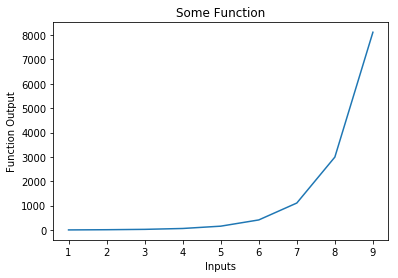Next, we want to be able to create variables as a part of our computational graph. Previously, when we defined a value with Tensorflow, it’s value couldn’t really be changed - it was specified as a tf.constant or specified once as an argument into feed_dict. As Adit discussed, with machine learning we want to be able to change the weights in our function over time as we train our model. The tf.Variable type helps us accomplish this!

### Creating Variables in Tensorflow

One way to create variables in tensorflow is with the tf.Variable() API. When creating variables, we usually want to provide a method of initializing the variable, and usually also want to mention the ** dimensionality ** of the variable (ie, do we wish to create a constant? Or do we wish to create a vector or matrix of a certain dimension?)

With Tensorflow, we need to explicitly initialize all of our variable by registering a variable initailizer, and running it within our session. This may sound a little complicated, but its just 2 lines of code.

W = tf.Variable(tf.constant(0.1, shape = [10,1])) # create a variable with information on how to initialize it and its dimensionality


Placeholders (tensors that we’ve promised to give data to later) can also take on arbitrary shapes. Of course, if you set a placeholder with a certain dimensionality, it will later expect data of that dimensionality!

x = tf.placeholder(tf.float32, shape = [1, 10]) # think of this as one training example that has 10 features.

mat_mul = tf.matmul(x, W)

data = np.arange(10).reshape((1,10)) # 0,1,2,3...10 reshaped into a 1 * 10 matrix

# initialize our variables
init = tf.global_variables_initializer() # the variable initializer
sess.run(init)
sess.run(mat_mul, feed_dict = {x: data}) # can you predict the output?

array([[ 4.5]], dtype=float32)


#### Exercise

Let’s create a function that takes in a set of 5 vectors each with dimensionality 10, and multiplies it with a matrix W of dimensionality $$10 * 5$$. What would we need to change in the code above?

# TODO: define a variable W_matrix to be a variable that is initialized with the constant 0.1, but has dimensionality 10 * 5
W_matrix = tf.Variable(tf.constant(0.1, shape = [10, 5]))
# TODO: define a variable x_matrix to be a placeholder of shape 5 * 10.
x_matrix = tf.placeholder(tf.float32, shape = [5, 10])
# TODO: redefine the above mat_mul operation to use W_matrix and x_matrix.
mat_mul = tf.matmul(x_matrix, W_matrix)

# TODO: define a new variable initializer, and run it.
init = tf.global_variables_initializer()
sess.run(init)


# TODO: create 50 arbitrary data points, and reshape them into a 5 * 10 matrix, so we can feed this into x as a placeholder.
data = np.arange(50).reshape((5, 10))
# TODO: run the function with sess.run(), and don't forget to pass in the argument for x_matrix!
ans = sess.run(mat_mul, feed_dict = {x_matrix: data})


### Recap

We’ve learned the very basics of tensorflow: how to represent the computational graphs, use placeholders to input data later, and create variables. It turns out that with these tools and a little knowledge about optimization in Tensorflow, we can easily implement many machine learning models. Let’s get to it!

### Linear Regression

Linear regression can be thought of as the “hello world” of machine learning. It’s relatively straigthforward to implement once you understand it, yet (unlike a simple “hello world” app) is powerful enough to have signficant use in industry. Let’s take a look at the linear regression problem.

We have some (input, output) pairs which we denote as $$(x_i, y_i)$$ and we have $$n$$ of these, so $$i \in [1...n]$$. We want to learn a function $$f: x \rightarrow{} y$$ that maps inputs to outputs.

Crucially, we want to learn the function $$f$$ such that $$f$$ generalizes well to unseen data. We can easily create a function $$f$$ that always returns the correct answer on data we’ve seen before (data in our training set) - can you guess what that function would look like, and why it would be essentially worthless for data we haven’t seen before?

### Today’s Dataset

We’ll be using the Boston house prices dataset. This dataset has 500 training examples, each with 13 features describing various attributes of the home, and a target associated with the example, giving the value of the home in thousands of dollars.

Each of the 13 features denotes some quality or quantity regarding the house or the surrounding area. A few examples of the different features included in the dataset include the area’s crime rate, the number of rooms in the house, the ** distance from the house to popular Boston locations, and the average **student to teacher ratio in nearby schools.

from boston_data_wrapper import get_data
X_train, X_test, y_train, y_test = get_data()

# TODO: what are the dimensionalities of our training and testing datasets and targets?
print(",".join([str(t.shape) for t in (X_train, X_test, y_train, y_test)]))

(354, 13),(152, 13),(354, 1),(152, 1)


As we’ve seen, we have 506 data points which we’ve split into two sets, X_train and X_test. We took 70% of the total data as training data (hence X_train), and 30% of the data as testing data (hence X_test). This is a popular split in machine learning - we always want to hold out some of our given data and not use it while training, so we have some data on which to evaluate the performance of our model.

The names y_train and y_test have a one-to-one correspondence to the values in X_train and X_test. In particular, for each house X_train[i], the value for the home is given by y_train[i].

To get more familiar with our dataset, let’s try to visualize how some of the features relate to the price of the house.

# get the crime rates and prices
crime_rates, prices = [X_train[i] for i in range(X_train.shape)], y_train.tolist()
# student-teacher ratio is the 10th feature
student_teacher_ratios = [X_train[i] for i in range(X_train.shape)]
# number of roots is the 5th feature
rooms = [X_train[i] for i in range(X_train.shape)]

plt.scatter(crime_rates, prices)
plt.xlabel('Crime Rate')
plt.ylabel('House Price')
plt.title('Crime Rates vs House Price')
plt.figure()
plt.scatter(student_teacher_ratios, prices)
plt.xlabel('Student Teacher Ratio')
plt.ylabel('House Price')
plt.title('Student teacher ratios vs House Price')
plt.figure()
plt.scatter(rooms, prices)
plt.xlabel('Rooms')
plt.ylabel('House Price')
plt.title('Number of rooms vs House Price')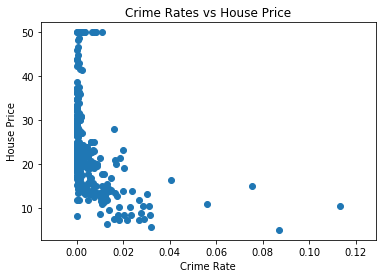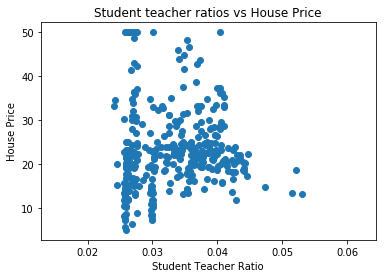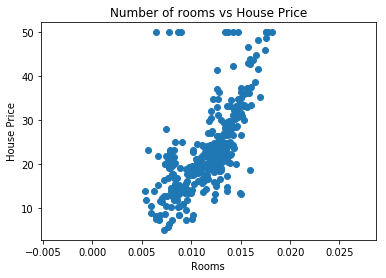#### Linear Regression: An overview

As discussed in the slides, we can teach a computer how to predict housing prices based on data. In order to do this, we will create a linear model in many dimensions (specifically, 13, the number of features in our dataset).

Our goal is to learn a function $$f: x \rightarrow{} y$$ that maps information about a house to the house’s price prediction. With linear regression, our function $$f$$ is just a linear combination of our inputs. That means our output is just the sum of our inputs, but each of our inputs are weighted by some value:

$f(x) = w_1 x_1 + w_2 x_2 + ... w_{13}x_{13} + b = \sum_{j=1}^{13} w_j x_j + b$

Next, we will initialize this linear model with initially random weights. As a result, our model won’t be able to predict house prices very well at all. Learning is the process of adjusting these parameters so that our model’s accuracy increases. In order to do this, we need to mathematically quantify how “bad” our model is currently. We can do this by calculating how off each prediction is from the actual value:

$L = \frac{1}{N} \sum_{i=1}^{N} (y_i - f(x_i))^2$

If we take the derivative of this function with respect to each of the weights $$w$$, we will know how much to “adjust” each weight $$w$$ by in order to make our function more accurate. This is an algorithm called gradient descent.

If you know some multivariable calculus, you can determine that the derivative with respect to the $$i$$th weight is $$\frac{dL}{dw_i} = \frac{-2}{N} \sum_{i=1}^{N} (y_i - f(x_i))x_i$$

This is getting a little abstract - lets move on to actually coding up this model!

# TODO: create placeholders for X and y, our features and tagets, respectively.
X = tf.placeholder(tf.float32, shape = [None, 13])
y = tf.placeholder(tf.float32, shape = [None,1])

# TODO: create variables for W and b, and initialize them with constants.
W = tf.Variable(tf.constant(0.1, shape = [13,1 ]))
b = tf.Variable(tf.constant(0.1))


# TODO: use Tensorflow to write out the linear regression model and assign it to a variable y_pred.
# y_pred = ...
y_pred = tf.matmul(X, W) + b
loss = tf.reduce_mean(tf.square(y_pred - y))

init = tf.global_variables_initializer()
sess = tf.InteractiveSession()
sess.run(init)
initial_loss = loss.eval(feed_dict = {X: X_train, y: y_train})
print("initial loss: {}".format(initial_loss))
for i in range(5000):
# TODO: run the optimization step with the training data passed in.
sess.run(opt, feed_dict = {X: X_train, y: y_train})
if i % 100 == 0:
# TODO: print the current error of the model so we can know how the model is doing while it is training
print("current loss: {}".format(loss.eval(feed_dict = {X: X_train, y: y_train})))

# TODO: evalute and print the final loss on the training and testing datasets.

initial loss: 605.6507568359375
current loss: 536.6200561523438
current loss: 66.02755737304688
current loss: 64.5899658203125
current loss: 63.52979278564453
current loss: 62.68644714355469
current loss: 61.97214889526367
current loss: 61.33811569213867
current loss: 60.756771087646484
current loss: 60.21210861206055
current loss: 59.69464874267578
current loss: 59.198455810546875
current loss: 58.71974182128906
current loss: 58.2559814453125
current loss: 57.80535888671875
current loss: 57.36661911010742
current loss: 56.93873596191406
current loss: 56.52097702026367
current loss: 56.11275100708008
current loss: 55.713531494140625
current loss: 55.32292175292969
current loss: 54.94056701660156
current loss: 54.5661506652832
current loss: 54.199440002441406
current loss: 53.84015655517578
current loss: 53.48813247680664
current loss: 53.14316177368164
current loss: 52.805023193359375
current loss: 52.473594665527344
current loss: 52.14869689941406
current loss: 51.830177307128906
current loss: 51.517913818359375
current loss: 51.211753845214844
current loss: 50.911563873291016
current loss: 50.617210388183594
current loss: 50.32859802246094
current loss: 50.04558563232422
current loss: 49.76805877685547
current loss: 49.49591827392578
current loss: 49.22903060913086
current loss: 48.96730041503906
current loss: 48.71063232421875
current loss: 48.458900451660156
current loss: 48.21202850341797
current loss: 47.96990966796875
current loss: 47.73243713378906
current loss: 47.499515533447266
current loss: 47.271080017089844
current loss: 47.047019958496094
current loss: 46.827247619628906
current loss: 46.6116828918457


Let’s evaluate our model and see how we did.

data, targets = X_test, y_test
predictions = sess.run(y_pred, feed_dict = {X: data})
predictions = predictions.flatten()
targets = targets.reshape((152))

# lets take a look at some predictions
for i in range(10):
randint = np.random.randint(0, 152)
pred = predictions[randint]
actual = targets[randint]
print("prediction: {}, actual was: {}".format(pred, actual))


prediction: 22.787330627441406, actual was: 21.7
prediction: 26.539813995361328, actual was: 25.0
prediction: 33.39194107055664, actual was: 28.5
prediction: 22.48349380493164, actual was: 21.8
prediction: 22.787330627441406, actual was: 21.7
prediction: 27.306413650512695, actual was: 50.0
prediction: 22.750877380371094, actual was: 21.5
prediction: 26.322635650634766, actual was: 19.4
prediction: 26.16617774963379, actual was: 23.6
prediction: 16.744220733642578, actual was: 9.7


Let’s plot the absolute differences as a function of the actual price. This will give us some intution around where our model is not so good - does it work equally well for all ranges of house prices, or does it perform worse depending on the actual house price?

diffs = abs(targets - predictions)
avg_diffs = np.mean(diffs)
avg_houseprice = np.mean(targets)
plt.xlabel('Home price values')
plt.ylabel('Diff btwn targets and functions')
plt.scatter(targets, diffs)
plt.show()
print("average absolute difference: {}".format(avg_diffs * 1000))
print("average house price: {}".format(avg_houseprice * 1000))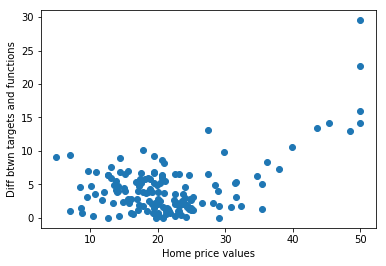average absolute difference: 4408.327848032901
average house price: 21407.894736842107


Our model seems to do okay with lower and averaged price houses, but appears to do terribly with higher-priced houses. Think about why this may be. It’s always important to consider the data that you used to train your model. In particular, it might be likely that our dataset didn’t have many examples of highly priced houses, so our model may have not learned how to predict prices for them. What’s cool about data science and machine learning is that we can easily test this theory.

All of the prices in the dataset are between 0 and 50 (in thousands of dollars). We can count up how many examples we have for houses between 0 and 10, 000, between 10,000 and 20,000, and so on. This will let us examine if there are any imbalances with respect to the house prices.

price_buckets = [0, 10, 20, 30, 40, 50]
limits = dict(zip(price_buckets, [0  for _ in range(len(price_buckets))]))
print(limits)
for price in targets:
limits[int(price/10) * 10]+=1

for k, v in sorted(limits.items()):
print("{} : {}".format(k, v))

{0: 0, 50: 0, 20: 0, 40: 0, 10: 0, 30: 0}
0 : 8
10 : 66
20 : 60
30 : 11
40 : 3
50 : 4


### Exercises

• As we have seen, our model is not very accurate for very highly-priced houses. How many higher-priced houses be different than lower-priced houses, and what did our model not capture about them?

• Investigate the need for a bias unit in our linear model. How can you adjust our current model to not have the bias unit (hint: just remove it). What happens to the model if the bias unit is removed? Why is having a bias unit important in machine learning models?

• Investigate different learning rates other than 0.5. Anything under 0.5 should work well, but you may have to play around with the number of iterations. Why is this - why do some learning rates require more iterations while others do not? Try learning rates greater than 0.5, and observe what happens. Why do you think this is? What do you think are some good guidelines to pick an optimal learning rate?

• Try Polynomial Regression. This involves generating additional features that are combinations of the original features. In higher-dimensional space, the house price and newly generated features may be linear with respect to each other. To do this, you’ll have to use the sklearn.preprocessing.PolynomialFeatures library to generate new features. Instead of 13, you’ll also have to update your model to take in the new number of features you have created.

• Do something interesting: If you have any ideas to change the model up or improve accuracy in some way, fork this repository and make your changes. Then post it on the AI at UCLA page for us to see! Also, don’t forget to create a pull request on our github if you want your change merged into our tutorials.

Thank you for attending! Please fill out our feedback form so we can produce even better content!

Written on October 13, 2017 by